# Mathematical understanding of RNN and its variants

• Difficulty Level : Hard
• Last Updated : 22 Jan, 2021

Introduction:

Experts expect Artificial Intelligence (AI) to work towards creating a better life to live. They say as more computation power will be available in the coming time i.e more graphical processing units, AI will make more advancement and productive to humans. Today, one can see a lot of such AI-powered applications like the fight against human trafficking, healthcare adviser, self-driving cars, Intrusion detection and prevention, object tracking and counting, face detection and recognition, disease prediction and virtual assistance for human help. This particular post talks about RNN, its variants (LSTM, GRU) and mathematics behind it. RNN is a type of neural network which accepts variable-length input and produces variable-length output. It is used to develop various applications such as text to speech, chatbots, language modeling, sentimental analysis, time series stocks forecasting, machine translation and nam entity recognition.

Table of content:

• What is RNN and how it is different from Feed Forward Neural Networks
• Mathematics behind RNN
• RNN variants (LSTM and GRU)
• Practical Applications of RNN
• Final Note

What is RNN and how it is different from Feed Forward Neural Networks:

RNN is a recurrent neural network whose current output not only depends on its present value but also past inputs, whereas for feed-forward network current output only depends on the current input.  Have a look at the below example to understand RNN in a better way.

Rahul belongs to congress.

Rahul is part of indian cricket team.

If anyone is asked who is Rahul, he/she will say that both Rahul is different i.e one is from Indian national congress and another is from the Indian cricket team. Now if the same task is given to the machine to give the output it cannot say until it knows the full context i.e predicting the identity of a single word depends on knowing the whole context. Such tasks can be implemented by Bi-LSTM which is a variant of RNN. RNN is suitable for such work thanks to their capability of learning the context. Other applications include speech to text conversion, building virtual assistance, time-series stocks forecasting, sentimental analysis, language modelling and machine translation.  On the other hand, a feed-forward neural network produces an output which only depends on the current input. Examples for such are image classification task, image segmentation or object detection task. One such type of such network is a convolutional neural network (CNN). Remember both RNN and CNN are supervised deep learning models i.e, they need labels during the training phase.

## Mathematics behind RNN

1.) Mathematical Equation of RNN

To understand the mathematics behind RNN, have a look at the below image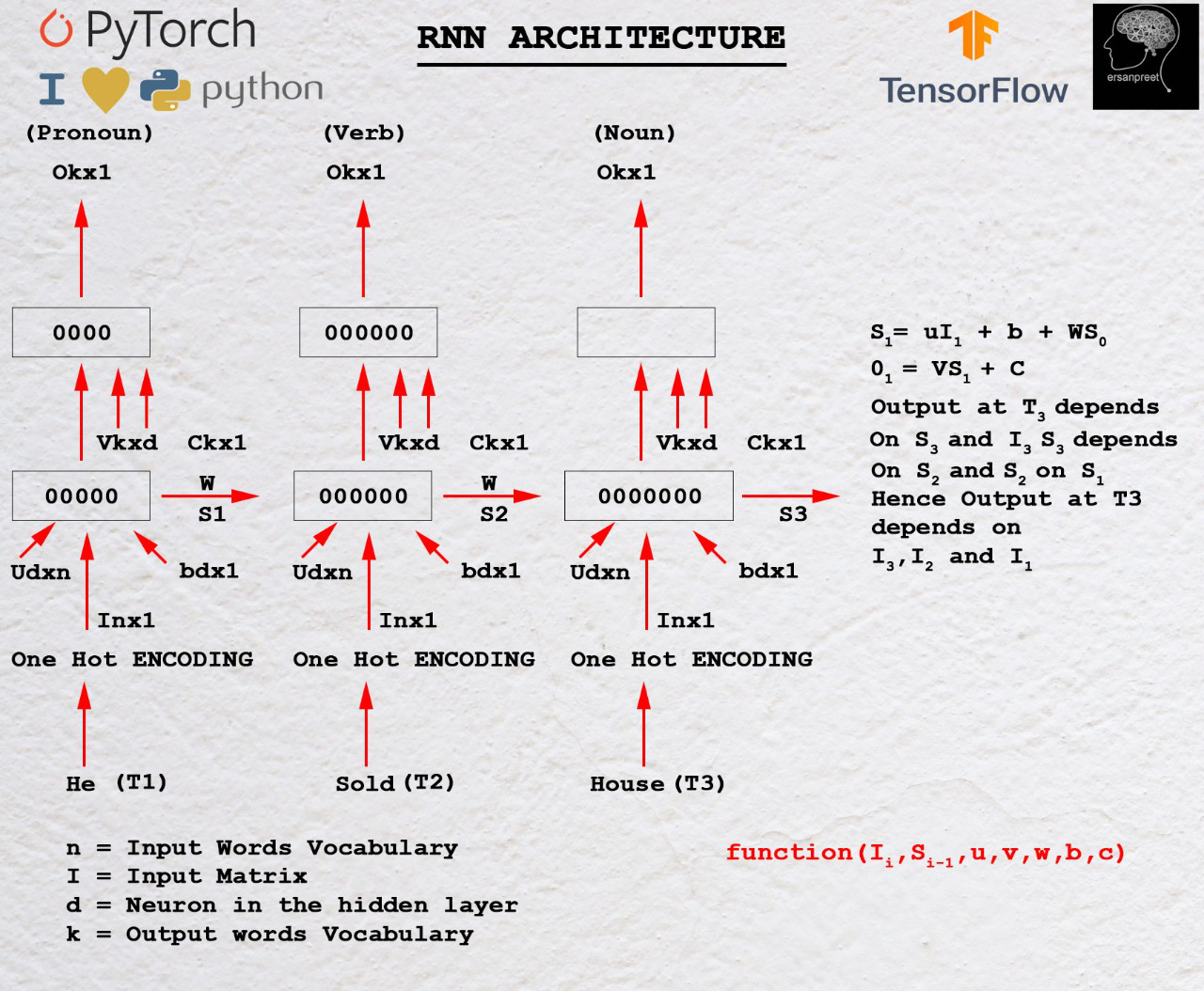Mathematics behind RNN

As discussed inside the first heading, output depends on both current and past inputs. Let I1 be the first input whose dimension is n*1 where n is the length of vocabulary. S0 be the hidden state to the first RNN cell having d neurons. For each cell, input hidden state should be one previous. For the first cell initialize S0 with zeros or some random number because no previous state is seen. U be another matrix of dimension d*n where d is the number of neurons in the first RNN cell and n is the input vocabulary size. W is another matrix whose dimension is d*d. b is bias whose dimension is d*1. For finding the output from the first cell, another matrix V is taken whose dimension is k*d where c is bias with dimension k*1.

Mathematically, outputs from the first RNN cell are as below

S1= UI1+ WS0 + b
O1= VS1+c

In General,

Sn= UIn+ WSn-1 + b
On= VSn+c

Key takeaway from the above equation

In general, output On depends on Sn and  Sn depends on Sn-1. Sn-1 depend on Sn-2.  Process goes till S0 is achieved.  This clearly demonstrates that output at the nth time step depends on all previous inputs.

### 2.) Parameters and Gradients

Parameters in the RNN are U, V, b, c, W  are shared among all the RNN cells. The reason for sharing is to create a common function which could be applied at all the time steps. Parameters are learnable and are responsible for training the model. At each time step, the loss is computing and is backpropagated through the gradient descent algorithm.

#### 2.1) Gradient of loss with respect to V

Gradient represents the slope of tangent and points in the direction of the greatest rate of increase of function. We are interested to find that V where loss is minimum. From the loss, it means cost function or error. In a simple sense, the cost function is the difference between a true value and predicted value. Move is made opposite to the direction of the gradient of the loss with respect to V. Mathematically new value of V is obtained using below mathematically formulaWhere d(L)/d(V)is the sum of all losses obtained from time steps. There are two ways of updating the weights. One is to calculate the gradient of the defined batch and then update it (Mini Batch) or calculate per sample and update (Stochastic). During the calculation of d(L)/d(V), the chain rule is applied. Have a look at the below figure to understand calculation and chain rule.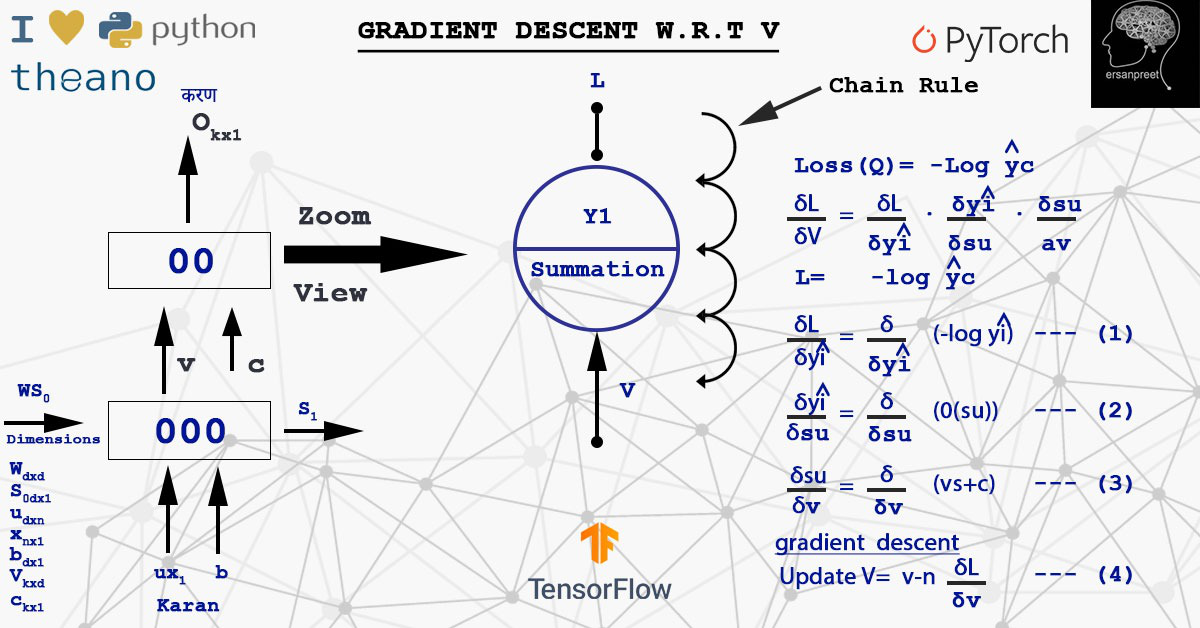Chain rule implementation for calculating gradient of loss w.r.t V

#### 2.2) Gradient of loss with respect to W

W is multiplied by S. In order to calculate derivative of loss with respect to weight at any time step, the chain rule is applied to take into consideration all the path to reach W from Sn to S0. This means that due to any of the wrong Sn, W is affected. In other words, some wrong information came from some hidden state which leads to loss. Mathematically, weight is updated as belowThe key point to remember is that either gradients and weights are updated at every sample or after a batch. This depends on algorithm one is choosing either stochastic or mini-batch. Have a look at the below screenshot to visualize the concept in a more refined way.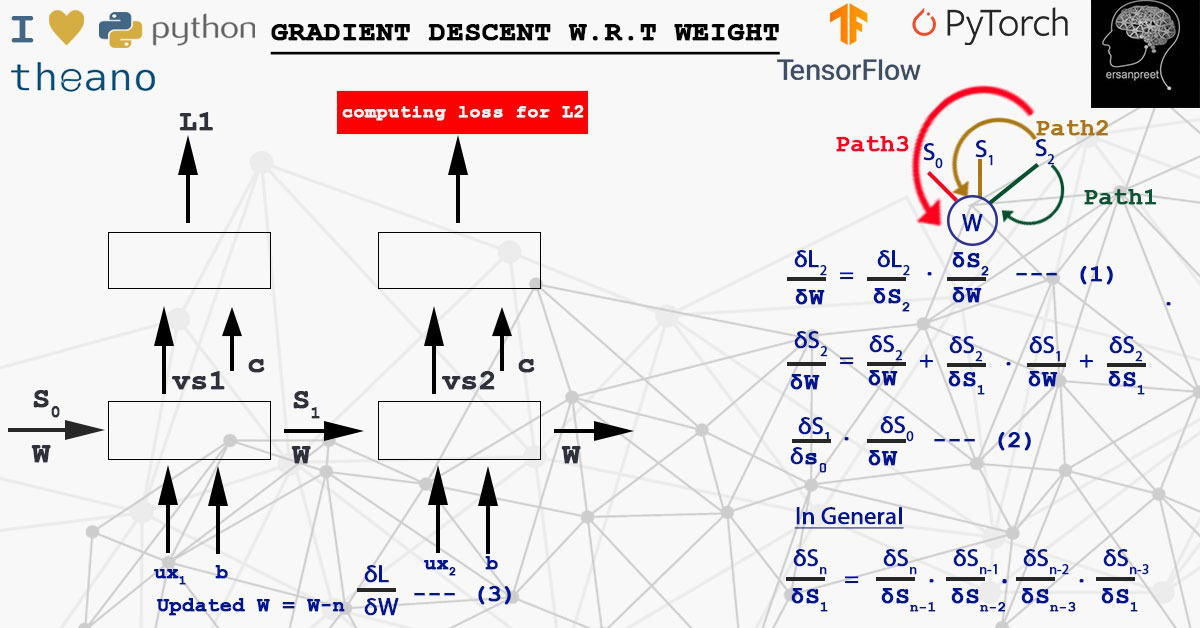Gradient descent with respect to W

## RNN variants (LSTM and GRU)

From the above discussion, I hope the mathematics behind RNN is clear now. The main drawback of RNN is whatever is the length of the sequence, the dimension of the state vector remains the same. Taking a case into consideration, if the length of the input sequence is very long, new information is being added to the same state vector. When one reaches the nth time step which is far away from the first time step, information is much confusing. At such a position, it is not clear what was the information provided at time step 1 or 2. It is analogous to a whiteboard whose dimension is fixed and one keeps on writing on it. At some position, it becomes very messy. One cannot even read what is written on board. To solve such issues, its variants were developed so-called LSTM and GRU. They work on the principle of selective read, write and forget. Now whiteboard (analogy to state vector) is same but only desired information is written at time step and unnecessary information is filtered out making sequential neural network suitable for training with long sequences. One can read the difference between LSTM and GRU from here.

### LSTM (Long Short Term Memory)

#### Mathematical Representation:

The strategy followed is selective write, read and forget.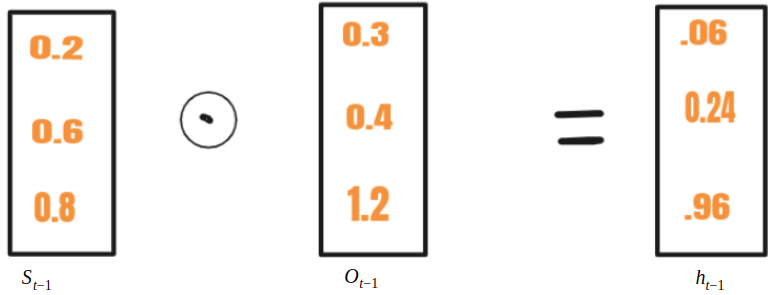Selective write

Selective Write:

In RNN,  St-1 is fed along with xt to a cell whereas in LSTM St-1 is transformed to ht-1 using another vector Ot-1. This process is called selective write. Mathematical equations for selective write are as belowHave a look at the below image to understand the concept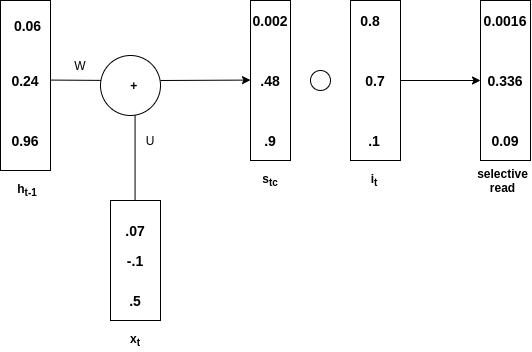ht-1 is added with xt to produce st. Then Hadamard product of(written stc in the diagram) and it is made to obtain st. This is called an input gate. In  st only selective information goes and this process is called selective read. Mathematically, equations for selective read are as belowSelective Forget:

Have a look at the below image to understand the concept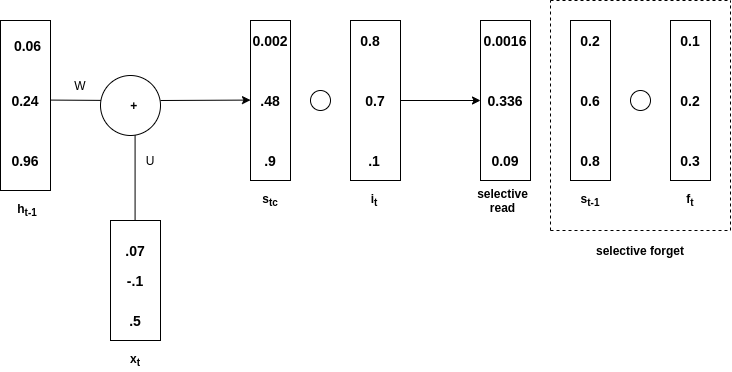Selective Forget

st-1 is hadamard product with ft and is called selective forget. Overall st is obtained from the addition of selective read and selective forget. See the below diagram to understand the above statement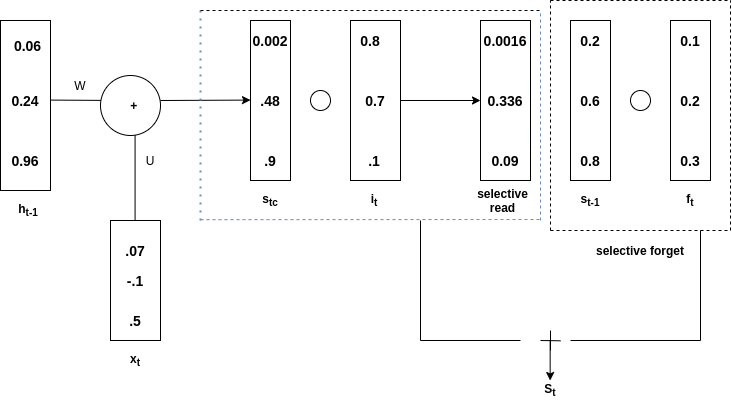Mathematically, equations for selective forget are as belowNote: There is no forget gate in case of GRU (Gated Recurrent Unit). It has only input and output gates.

Practical Applications of RNN:

RNN finds its use case in a speech to text conversion, building virtual assistance, sentimental analysis, time series stocks forecasting, machine translation, language modelling. More research is going on creating generative chatbots using RNN and its variants. Other applications include image captioning, generating large text from a small paragraph and text summarizer (an app like Inshorts is using this). Music composition and call centre analysis are other domains using RNN.

Final Note:

In a nutshell, one can understand the difference between RNN and feed-forward neural network from the opening paragraph and then going deep into the mathematics behind RNN. In the end, the article is completed by explaining different variants of RNN and some practical applications of RNN. In order to work on applications of RNN, one must gain strong knowledge in calculus, derivatives especially how chain rule works. Once the theory is studied, some codes on these topics should be made in your favorite coding language. This will provide you with the upper hand.

My Personal Notes arrow_drop_up
Recommended Articles
Page :